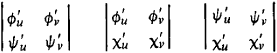# surface

(redirected from impression surface)
Also found in: Dictionary, Thesaurus, Medical, Legal.
Related to impression surface: impression surface of denture

## surface

1. Geometry
a. the complete boundary of a solid figure
b. a continuous two-dimensional configuration
2.
a. the uppermost level of the land or sea
b. (as modifier): surface transportation

## Surface

a fundamental geometric concept with different meanings in different branches of geometry.

(1) A high-school geometry course considers planes, polyhedrons, and some curved surfaces. Each of the curved surfaces is defined in a special way— most often as a set of points that satisfy certain conditions. For example, the surface of a sphere is the set of points at a specified distance from a given point. The concept of a surface is merely exemplified rather than defined. Thus, a surface is said to be the boundary of a solid or the trace of a moving curve.

(2) The mathematically rigorous definition of a surface is based on the concepts of topology. The principal concept here is that of a simple surface, which may be represented as a part of a plane that is subject to continuous deformation— that is, to continuous extension, compression, or bending. More precisely, a simple surface is the image of the interior of a square under a homeomorphic, that is, a one-to-one and bicontinuous, mapping. This definition can be expressed analytically as follows. Introduce Cartesian coordinates u, v in the plane and x, y, z in space. Let S be the (open) square whose points have coordinates satisfying the inequalities 0 < u < 1 and 0 < v < 1. A simple surface is the homeomorphic image in space of the square Sʹ. The surface is given by means of formulas x = Φ (u, v), y = ψ(u, v), z = x(u, v), which are called its parametric equations. For different points (u, v) and (u ʹ, vʹ) the corresponding points (x, y, z) and (xʹ, yʹ, zʹ) must be different, and the functions Φ(u, v), ψ(u, v), and x(u, v) must be continuous. The hemisphere is an example of a simple surface. The sphere, however, is not a simple surface. Further generalization of the concept of a surface is consequently necessary. If a neighborhood of each point of a surface is a simple surface, the surface is said to be regular. From the standpoint of topological structure, surfaces as twodimensional manifolds are divided into several types, such as closed and open surfaces and orientable and nonorientable surfaces.

The surfaces investigated in differential geometry usually obey conditions associated with the possibility of using the methods of the differential calculus. These are usually smoothness conditions, such as the existence of a tangent plane or of curvature at each point of the surface. These requirements mean that the functions Φ(u, v), ψ(u, v), and x (u, v) are assumed to be once, twice, three times, or, in some problems, infinitely differentiable or even analytic. Moreover, it is required that at each point at least one of the determinantsbe nonzero.

In analytic and algebraic geometry, a surface is defined as a set of points whose coordinates satisfy an equation of the form

(*) Φ(x, y, z) = 0

Thus, a given surface may or may not have a graphic geometric image. In this case, in order to preserve generality, we speak of imaginary surfaces. For example, the equation

X2 + y2 + z2 + 1 = 0

defines an imaginary sphere, although real space contains no point with coordinates satisfying this equation. If the function Φ(x, y, z) is continuous at some point and has at this point continuous partial derivatives ∂Φ/ ∂x, ∂Φ/ ∂y, ∂Φ/∂z, at least one of which does not vanish, then in the neighborhood of this point the surface defined by equation (*) will be a regular surface.

## surface

[′sər·fəs]
(engineering)
The outer part (skin with a thickness of zero) of a body; can apply to structures, to micrometer-sized particles, or to extended-surface zeolites.
(mathematics)
A subset of three-space consisting of those points whose cartesian coordinates x, y, and z satisfy equations of the form x = ƒ(u, v), y = g (u, v), z = h (u, v), where ƒ, g, and h are differentiable real-valued functions of two parameters u and v which take real values and vary freely in some domain.

## surface

(1) (Surface) Microsoft's hardware brand. See Surface versions.

(2) In CAD, the external geometry of an object. Surfaces are generally required for NC (numerical control) modeling rather than wireframe or solids.
References in periodicals archive ?
Other advantages to this technique include easy retrieval of the model from the three layers of impression surface, easy retrieval of the wax pattern prepared from the impression surface of the donor technique, and less voids while recording the impression of the internal surface of the external ear.
Examine the impression surface for surface irregularities by using disclosing material to identify the position and extent of over-contour and relieve appropriately.
The three surfaces are: (1) the impression surface (in contact with the tissues), (2) the occlusal surface (in contact with the opposing teeth or denture), and (3) the polished surfaces (5) (consisting of labial, buccal and lingual surfaces).

Site: Follow: Share:
Open / Close